Orginal Article

# 二维材料力学行为的压痕测试

1 北京大学应用物理中心, HEDPS, 北京 100871
2 同济大学航空航天与力学学院, 上海 200092
3 上海交通大学IFSA 协同创新中心, 上海200240

# Indentation tests investigation of mechanical behavior of two-dimensional materials

ZHOU Lixin1, CAO Guoxin23ɛ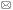1 HEDPS, Center for Applied Physics and Technology, Peking University, Beijing 100871, China
2 School of Aerospace Engineering and Applied Mechanics, Tong ji University, Shanghai 200092, China
3 IFSA Collaborative Innovation Center, Shanghai Jiao Tong University, Shanghai 200240, ChinaAbstract

Fully understanding the mechanical properties of two-dimensional (2D) materials is highly important for developing applications based on 2D-materials. Free-standing indentation (FSI) is currently the most common method to measure the mechanical properties of 2D-materials. The present work reviewed the state-of-the-art FSI investigations of 2D-mateirals, the issues to be considered, and some future works in this area. In FSI tests, 2D-materials are firstly transferred on the top of the substrate with cylindrical holes to create beam/drum-type samples, and atomic force microscopy (AFM) is then used to measure the indentation load-displacement relationship of these samples. Finally, the mechanical properties, including the elastic modulus and intrinsic strength, can be determined by fitting the experimental results as the indentation analysis model is developed on the basis of the continuum thin film. However, since the thickness of 2D-materials is far less than that of the continuum thin film, the van der Waals (vdW) adhesion interactions from the AFM-tip and the side-wall of substrate hole have a strong influence on the indentation response, which leads to measurement inaccuracy of the elastic modulus of 2D-materials from FSI tests. In addition, the nonlinear response of 2D-materials under large deformation as well as the stress concentration created by defects cannot be effectively described by the conventional indentation analysis model, and thus, the intrinsic stress of 2D-materials cannot be accurately determined, especially for the poly-crystalline 2D-materials. Therefore, we should correctly understand the present experimental results from FSI tests, and in the meantime, it is very necessary to further improve the FSI technique for measuring the mechanical properties of 2D-materials.

Keywords： two-dimensional materials ; elastic modulus ; intrinsic strength ; atomic force microscopy ; indentation tests

0

ZHOU Lixin, CAO Guoxin. Indentation tests investigation of mechanical behavior of two-dimensional materials[J]. Advances in Mechanics, 2018, 48(0): 1804- https://doi.org/10.6052/1000-0992-16-043

## 2 无基底压痕测试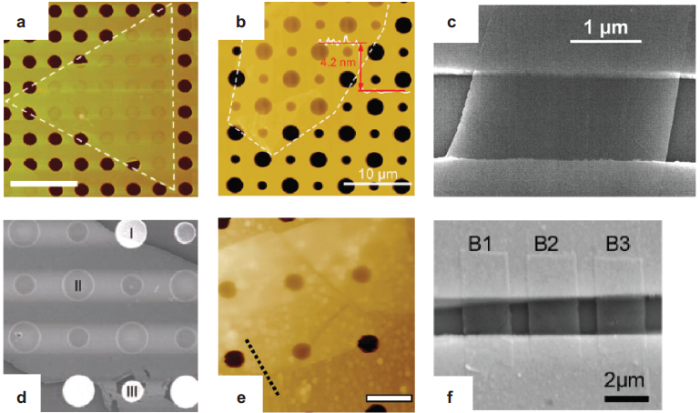### 2.1 两端固支的二维"梁"模型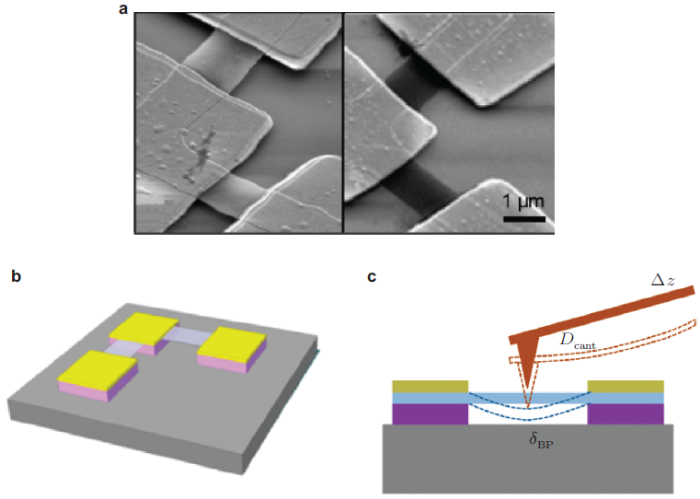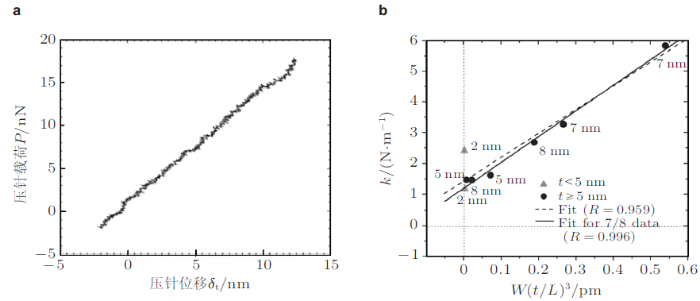$k = \dfrac{P}{\delta _{\rm t} } =\dfrac{L}{12\alpha }\dfrac{E}{\left( {1 - \nu ^2} \right)}\left({\dfrac{t}{L}} \right)^3 \ \ (6)$其中, $$\alpha$$值取决于薄膜的长宽比$$L/W$$和边界条件, 对于两端简支板$$L/W =1$$, $$\alpha =0.0116$$; 对于两端固支板$$L/W =1$$, $$\alpha =0.00724$$, 通过式(6), Kunz等 (2009)测量得出细黏土薄层$$(t<25$$ nm)的弹性模量为$$E= (21\pm 9)$$ GPa.

### 2.2 周边固支的二维"鼓"模型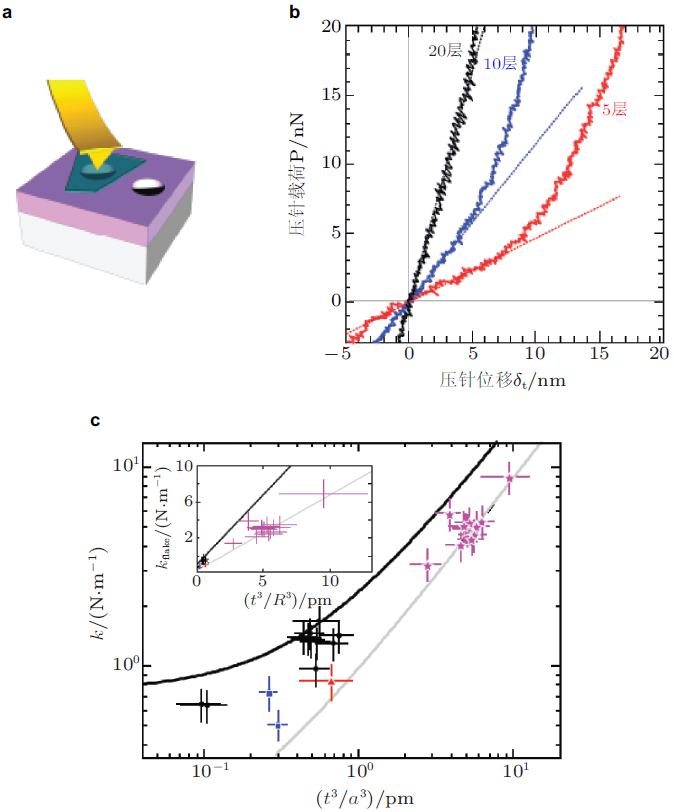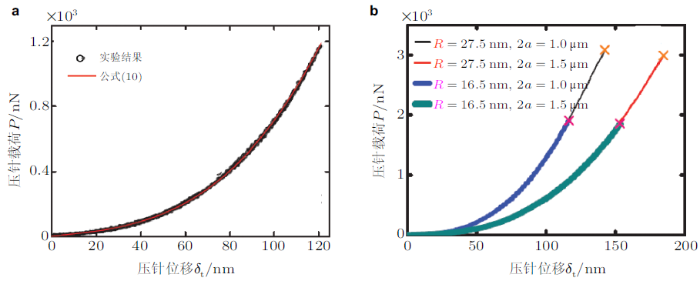Ruiz-Vargas等 (2011)认为, 当薄膜存在预拉伸时, 薄膜的压针载荷$$P$$和压针的尺度相关, 他们将压针半径$$R$$引入到$$P$$-$$\delta _{\rm t}$$关系的线性项中, 给出一个与式(10)略有差异的$$P$$-$$\delta _{\rm t}$$关系模型$P = \dfrac{2\pi \sigma _0 t\delta _{\rm t} }{\ln\left( {a / R} \right)} + Et\xi ^3a\left( {\dfrac{\delta _{\rm t}}{a}} \right)^3 \ \ (12)$一般情况下, 由于$$a/R >10$$, 如$$a/R$$为$$18\sim 45$$ (Lee et al. 2008), 与式(10)相比, 由式(12)拟合出的$$\sigma _{0}$$值(薄膜预应力)更大, 而且这种差别随着$$a/R$$的增大变得更为明显.

## 3 压痕响应分析模型的有效性

### 3.1 无基底二维材料的边界条件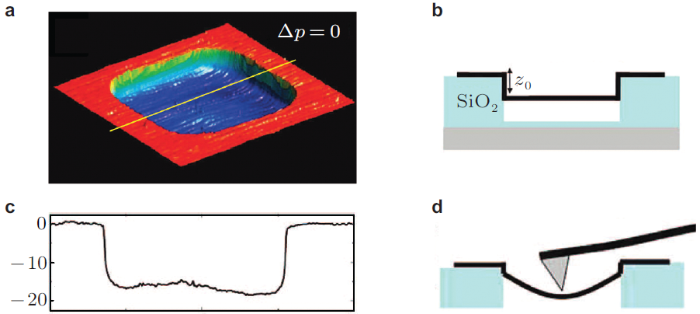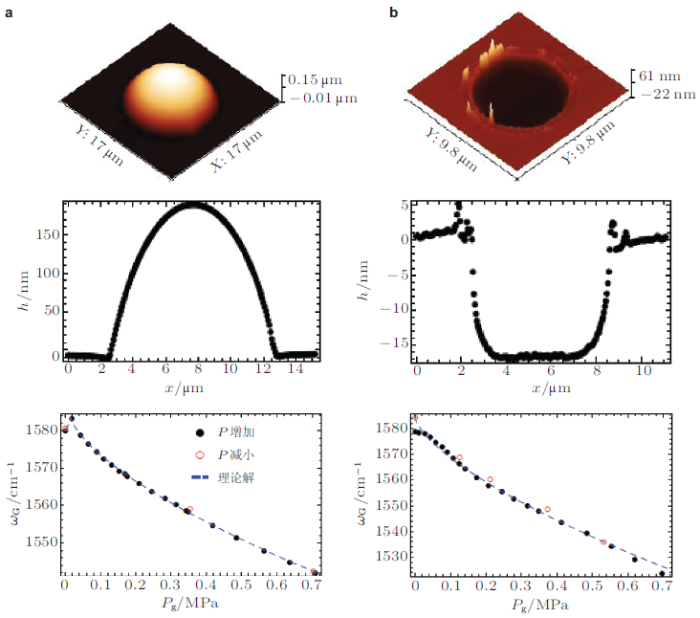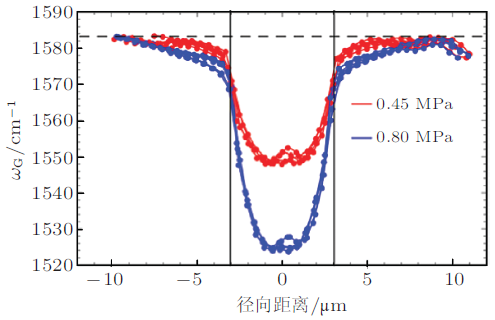Lin等 (2013)通过AFM测量了覆盖在基底柱状孔上石墨烯的形貌变化情况, 包括初始表面形貌, 小压痕载荷 (约100 nN)、大压痕载荷(约1 000 nN)作用后的形貌以及相对应的中心割线位置的高度变化, 如图9 所示. 在初始状态 (图9(a))时, 小部分石墨烯贴附在界面上, 悬空部分存在一定的弯曲, 并非完全平直; 在小压痕载荷作用后(图9(b)), 黏附的石墨烯部分从侧壁上剥离, 悬空部分向上弯曲, 弯曲程度大于图9(a); 在较大压痕载荷作用后 (图9(c)), 悬空部分的石墨烯呈现出一种类似于薄壳的压缩失稳状态 (上下波动起伏).上述结果再次印证石墨烯与基底柱形孔侧壁的贴附强度很弱, 小载荷作用下即可以发生与侧壁之间的剥离.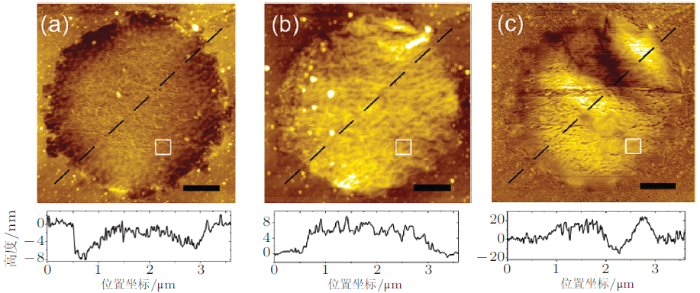Zhou等 (2013d)通过MD模拟进一步证实了上述实验结果, 在模拟中采用类似于实验中观测到的石墨烯几何结构 (中心部分悬空, 在范德华作用下侧边贴附在SiO$$_{2}$$孔壁上, 如图10 所示).模拟结果显示, 石墨烯悬空部分在集中力作用下, 首先是贴附在侧壁的石墨烯开始剥离, 直到完全剥离后, 悬空部分石墨烯的应变才随着压针位移的增大而上升, 而且上述模拟结果对石墨烯侧壁贴附长度以及悬空部分长度都不敏感.Zhou等 (2013d)指出, 虽然石墨烯和基底之间的贴附能可以使石墨烯和基底紧密贴附(石墨烯和SiO$$_{2}$$的结合能为0.1 J/m$$^{2})$$, 但是在剥离过程中, 石墨烯并非沿着侧壁方向整体滑动分离, 而是在近似垂直方向作用力下一层层分离, 即每次外力作用仅需要克服接触分界线上一层碳原子的范德华能, 因此极低的面内应力就会造成薄膜从侧壁剥离.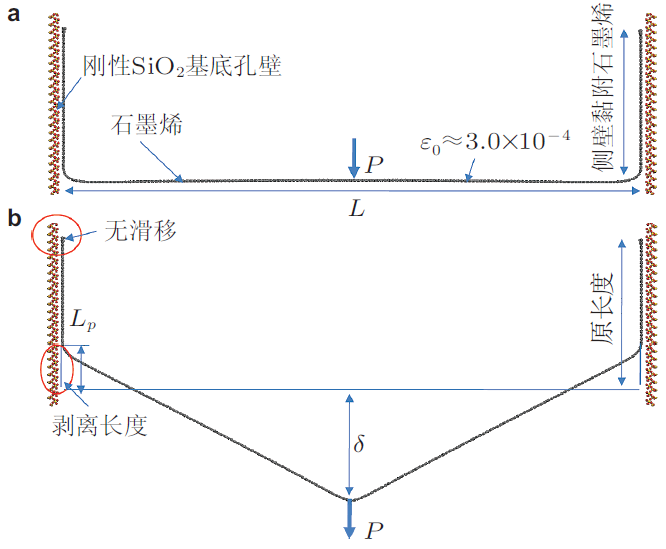### 3.2 AFM压针与无基底二维材料之间的范德华作用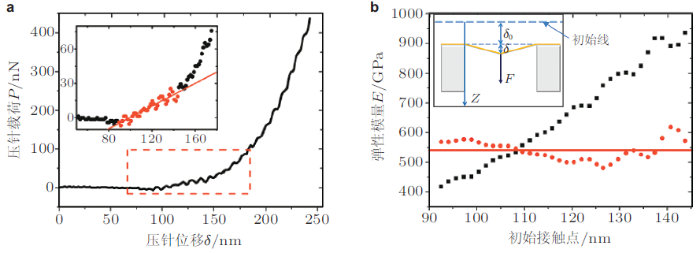### 3.4 压痕响应模型有效性的计算模拟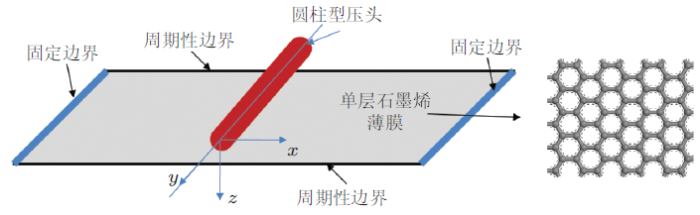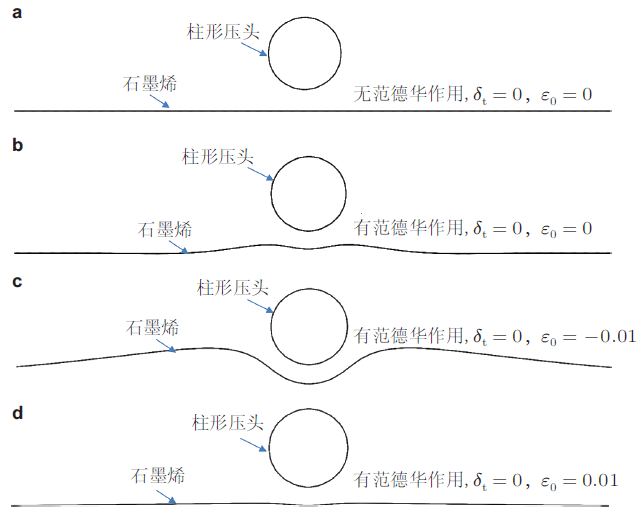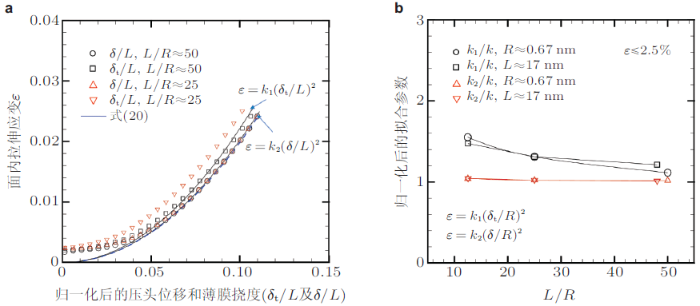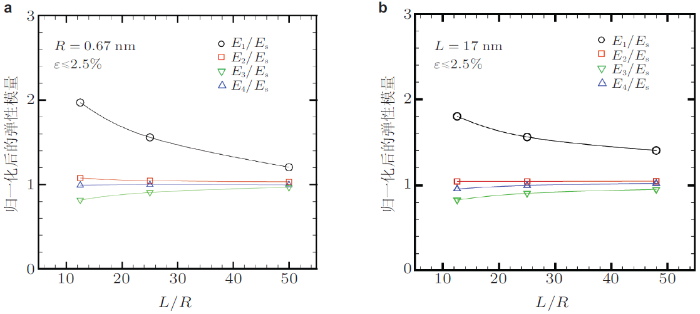Zhou等(2013d) 通过模拟无预应力石墨烯发现, 虽然获得的压痕载荷--压针位移关系不能很好地符合式(23), 却可以很好地符合式(26), 而且可以拟合出一个有效预拉伸应变$$(\varepsilon _{0})$$, 这和一些已报道的石墨烯无基底压痕响应MD模拟结果相同 (Neek-Amal & Peeters 2010, 杨晓东等 2010, Tan et al. 2013). Zhou等(2013d)指出, $$\delta _{\rm t}$$和$$\delta$$的差异正是可以拟合出预拉伸的根本原因, 这是一个由拟合产生的"伪"预应力, 显然不能反映薄膜的真实预应力状态. 事实上, 通过比较式(22)和式(23)可以很容易地推导出上述"伪"预应变$\varepsilon _0 = \dfrac{k\left( {\delta ^3 - \delta_{\rm t} ^3} \right)}{L^2\delta _{\rm t} } \ \ (27)$因此在$$\delta _{\rm t} \neq\delta$$情况下, $$\delta _{\rm t}<\delta$$对应预拉伸应力, 而$$\delta _{\rm t}>\delta$$对应预压缩应力. 在目前的无基底压痕测试中, 无论是基于"梁"模型还是"鼓"模型, 均假设样品最大挠度和压针位移值相等 (即$$\delta \approx \delta _{\rm t})$$, 对于厚度 $$(t)$$较大的连续介质薄膜, 上述假设相对有效, 但对于$$t$$值较小的二维材料, 上述假设并不有效(特别是单、双层二维材料), AFM压针和二维材料之间的范德华吸引作用会对初始接触点的确定有明显影响(Lee et al. 2008, Lin et al. 2013), 导致$$\delta _{\rm t}\neq\delta$$, 进一步造成了"伪"预应力的出现.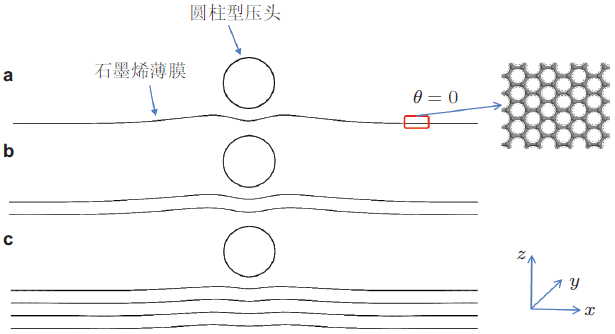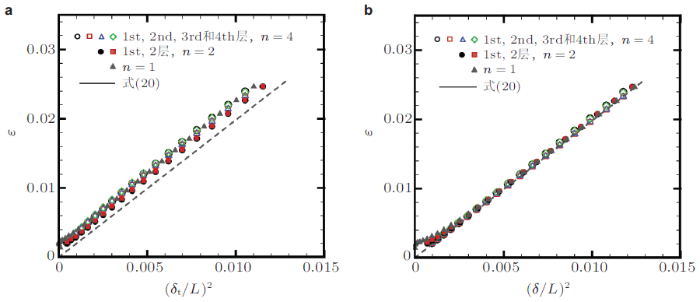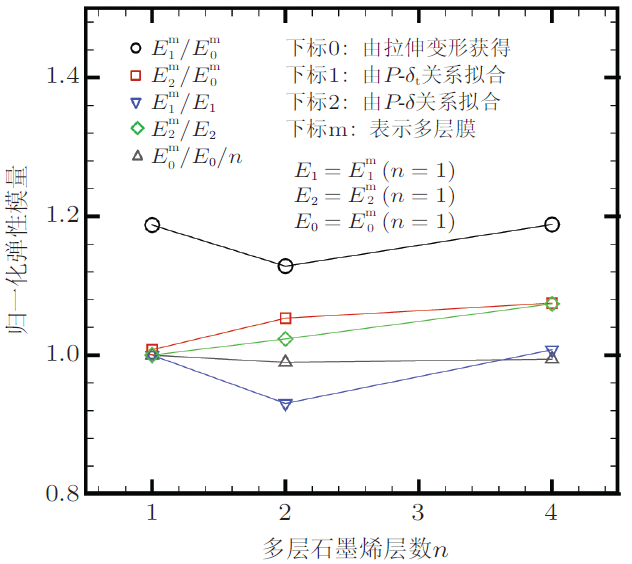## 4 二维材料强度的压痕模型有效性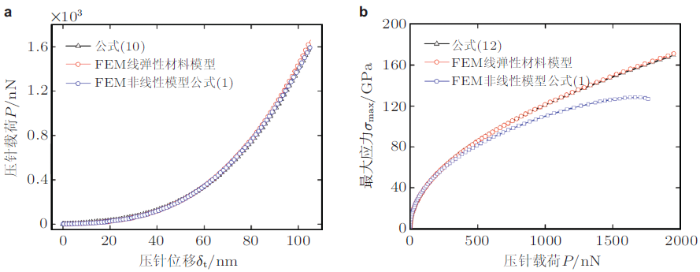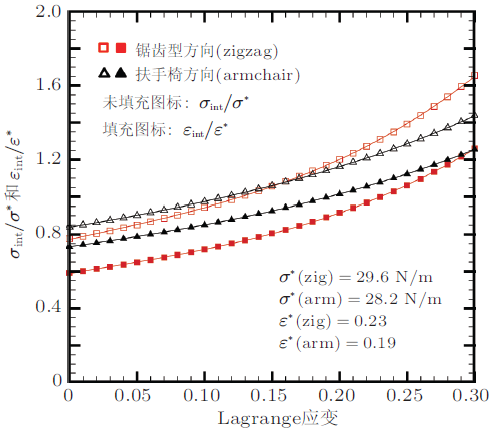## 5 多晶二维材料的压痕有效性

Lee等(2013)通过无基底压痕实验分别测量了单晶石墨烯、小尺度晶粒多晶石墨烯(1 $$\sim$$ 5 $$\muup$$m)和大尺度晶粒多晶石墨烯 (50 $$\sim$$ 200 $$\muup$$m)的力学性能, 发现, 不仅所有石墨烯的弹性模量非常接近, 多晶石墨烯比单晶$$E$$值最多高出5%, 而且它们的断裂强度也非常接近(98.5 $$\sim$$ 103 GPa), 小尺度晶粒的强度只比大尺度晶粒与单晶石墨烯最多低5%); 他们还通过AFM压针直接压在晶界上测量了晶界强度与晶界错配角之间的关系.结果显示, 晶界的断裂载荷只比单晶石墨烯低20% $$\sim$$ 40%(远高于Ruiz-Vargas等报道的结果), 而且其强度对晶界错配角(晶界两侧晶粒的取向差)并不敏感. Lee等(2013)认为能得到与单晶石墨烯近似的弹性模量的原因是: 多晶样品质量较好, 没有褶皱, 因而可进一步认为晶界的出现并不能显著影响石墨烯的弹性模量(前提是不产生褶皱); 另外, 他们也分析了晶界强度与晶界错配角无关的原因, 认为由于实验中多晶石墨烯的晶界远比计算模拟中的双晶晶界复杂, 晶界产生的预应力会被释放 (如大角度晶界), 导致较高的晶界强度以及对晶界角的不敏感性. Rassol等(2013)测量了多晶石墨烯晶界强度与晶界的错配角的关系, 发现, 具有大角度晶界的双晶 (无论是凹形还是锯齿形方向)的晶界强度 $$(\sigma_{\max}$$为$$80\sim 83$$ GPa)略低于单晶强度 (90 $$\sim$$ 94 GPa); 但小角度晶界双晶的晶界强度要明显低于其单晶强度, 而且还有较大的波动$$(\sigma _{\max}$$为$$53\sim 77$$ GPa), 这些结果与计算模拟结果吻合较好 (Wei et al. 2012).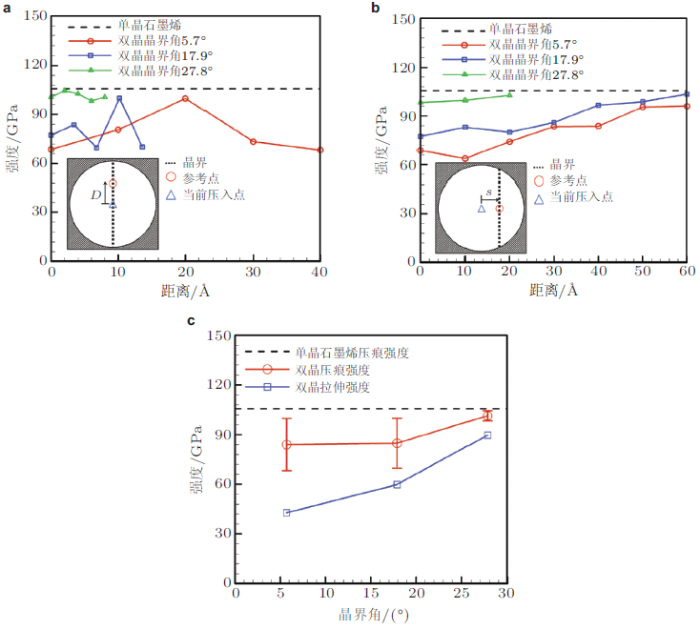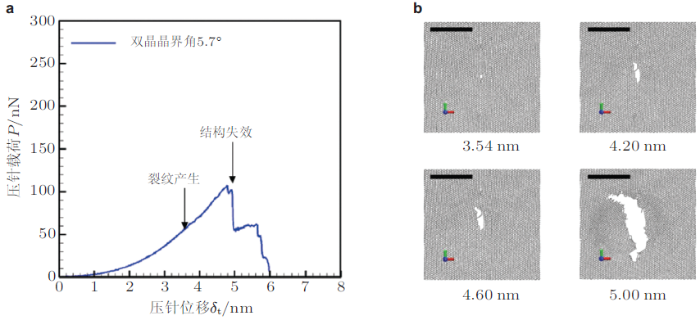## 6 二维材料无基底压痕研究展望

(1)目前所使用的二维材料无基底压痕响应分析模型均是基于连续介质薄膜推导出的, 模型中采用的固支边界条件、压针位移近似等于薄膜最大挠度等重要假设对于连续介质薄膜是有效的, 但对于二维材料来说可能并不有效, 这主要由于二维材料的厚度远小于连续介质薄膜, 因此无论是来自AFM压针还是基底孔侧壁的范德华相互作用对无基底二维材料的形貌均有显著影响.首先, 需要通过计算模拟来估算其对所获得力学性能的影响, 及其与系统参数 (压针半径、基底孔径和压针位移等)之间的关系, 优化测试条件, 如适当的加大压针位移可以有效降低范德华作用的影响(Lin et al. 2013; Zhou et al. 2013e, 2013c); 其次, 采取必要措施降低范德华相互作用对力学性能测试的影响, 如引入真实的预拉应力, 可以降低基底孔侧壁与压头范德华作用的影响(Zhou et al. 2013); 或者引入硬度较小的基底材料, 通过加入基底和二维材料之间的范德华作用抵消AFM压针范德华作用的影响(Niu et al. 2016).

(2)目前所报道的二维材料无基底压痕实验条件范围变化非常大, 例如: 基底孔半径$$a$$为$$0.275\sim 1.9 \muup$$m、材料厚度$$t$$为$$0.335\sim14.3$$ nm (从单层石墨烯到多层黑磷)、压针半径$$R$$为$$8\sim115$$ nm、压针位移$$\delta _{\rm t}$$为$$20\sim 130$$ nm, 但均采用了统一的压痕分析模型.而且通常采用的无基底压痕模型是假设受集中载荷作用并忽略薄膜抗弯刚度导出的, 而这些假设所引起的误差在上述范围内有较大的不同, 因此, 需要通过计算模拟给出通用压痕模型相对于上述系统参数, 如薄膜和压头半径比$$a/R$$、压针位移和薄膜半径比$$\delta _{\rm t}/a$$等的误差分析, 以确定合理的实验条件.

(3)由于球形压针在压入点附近会产生明显的应力集中, 而材料的断裂失效对应力集中又非常敏感, 这将导致由无基底压痕测试获得的二维材料破坏强度要低于其平面拉伸强度, 特别是对于内部有缺陷的二维材料 (含有晶界的多晶二维材料), 这种影响会进一步强化. 另外, 对于多晶二维材料, 压入点的选择很可能会影响强度的测量值, 过于靠近晶界会引起应力集中耦合效应, 导致强度测量值显著低于其真实强度; 而过于远离晶界, 又会由于压痕变形随着远离压入点而迅速衰减, 使得晶界处在变形中并不开裂, 导致测量强度显著高于其真实强度.

(4)目前计算模拟对理解二维材料力学行为的帮助还很有限, 特别是关于材料的无基底压痕响应, 还无法与实验结果进行有效对照, 这主要是由于计算模拟大多是基于MD模拟, 还无法进行真实尺度的压痕模拟(如真实的压针、石墨烯尺度), 也无法给出真实的压痕应力分布, 因为应力分布不仅取决于石墨烯和压针尺度比$$a/R$$, 还直接取决于压针尺度$$R$$, 这对于模拟石墨烯的破坏非常重要, 因此需要进一步发展多尺度模拟. 另外, 在计算模拟中需要更准确地描述二维材料无基底压痕的真实边界条件(靠近基底孔壁的部分二维材料依靠范德华作用黏附在孔壁上), 以及考虑二维材料在加载前的几何形貌 (具有一定褶皱度), 这样才能更好与实验结果对照.

(5)在无基底压痕计算模拟中对由压针或缺陷(晶界)引起应力集中以及最终破坏应力的定量表征还缺乏有效的方法.首先, 目前由MD模拟获得的缺陷应力是在原子尺度(即每个缺陷原子都有相应的应力值), 如何和宏观连续介质定义的缺陷应力挂钩还没有明确的解决方案, 如是否需要定义一个有效范围对原子尺度的缺陷应力进行平均化之后再与宏观应力相关联, 以及如何定义这个有效范围. 其次, 根据实验测量, 二维材料的本征强度由最大压痕载荷确定, 而在MD计算模拟中, 原子之间的化学键断裂是由成键原子间距控制的, 石墨烯中C-C间距如果大于0.192, 就被认为该键发生断裂 (Wei et al. 2012), 而当键发生断裂时, 压痕载荷仍可以继续增大, 因此如何通过计算模拟确定二维材料的压痕强度仍不明确.

## 7 结语

(1)由于二维材料的厚度远小于连续介质薄膜, 范德华作用(压针--二维材料以及二维材料--基底孔侧壁)对二维材料有比较显著的影响, 压针与二维材料之间范德华作用会使得初始接触位移零点很难确定, 而且压针位移$$\delta _{\rm t}$$和二维材料的最大挠度$$\delta$$并不相等$$(\delta _{\rm t}<\delta )$$, 这种差异会引起二维材料弹性模量被高估; 二维材料与基底孔侧壁之间的范德华作用, 造成在孔侧壁黏附的二维材料部分在压痕变形过程中从侧壁剥离, 导致弹性模量被显著低估.

(2)二维材料与基底孔侧壁之间的范德华作用非常弱, 无法形成固支边界条件, 也无法在薄膜内产生初始预拉伸应力, 而压痕实验中获得的预拉伸应力值是由$$\delta _{\rm t} \neq\delta$$引起的拟合误差产生的, 是一种"伪"预应力, 在MD计算模拟中, 从无初始预应力的二维材料的压痕响应中也可以拟合出一个预拉伸应力值, 但由于这个拟合预拉伸项的出现, 由$$\delta _{\rm t} \neq\delta$$引起的弹性模量的高估一定程度被降低了.

(3)在通过最大压痕载荷测量二维材料的本征强度时, 由于在达到破坏时, 二维材料具有很强的材料非线性效应, 因此采用由线弹性模型得出的最大压痕载荷和薄膜最大应力之间的关系, 将会明显高估二维材料的本征强度; 而且, 由于二维材料非线性模型$$(\sigma = E\varepsilon + D\varepsilon^2)$$中的$$D$$值随变形的增大而逐渐减小, 采用该模型由有限元反向分析获得的本征强度也会明显高估二维材料强度.

(4)压针在压入点附近引起应力集中, 而其中缺陷(晶界)也会引起应力集中, 当压入点远离缺陷 (晶界)时, 缺陷对压痕强度无影响, 而压入点靠近缺陷时, 会导致应力集中耦合效应, 压痕强度显著降低.这些因素导致了目前通过压痕实验测量的多晶石墨烯强度值的巨大波动, 因而无法准确反映晶界强度.

The authors have declared that no competing interests exist.

## 参考文献 原文顺序 文献年度倒序 文中引用次数倒序 被引期刊影响因子

  韩同伟, 贺鹏飞, 骆英, 张晓燕. 2011. 石墨烯力学性能研究进展. 力学进展, 41: 279-293  (Han T W, He P F, Luo Y, Zhang X Y.2011. Research progress in the mechanical properties of graphene. Advances in Mechanics, 41: 279-293).  杨晓东, 贺鹏飞, 吴艾辉, 郑百林. 2010. 石墨烯纳米压痕实验的分子动力学模拟. 中国科学: 物理学力学天文学, 40: 353-361  (Yang X D, He P F, Wu A H, Zheng B L.2010. Molecular dynamics simulation of nanoindentation for graphene. Scientia Sinica,40: 353-360).  Annamalai M, Mathew S, Jamali M, Zhan D, Palaniapan M.2012. Elastic and nonlinear response of nanomechanical graphene devices. Journal of Micromechanics and Microengineering, 22: 105024.  Arroyo M, Belytschko T.2004. Finite crystal elasticity of carbon nanotubes based on the exponential Cauchy-Born rule. Physical Review B, 69: 115415.  Balandin A A, Ghosh S, Bao W Z, Calizo I, Teweldebrhan D, Miao F, Lau C N.2008. Superior thermal conductivity of single-layer graphene. Nano Letters, 8: 902-907.  Bao W X, Zhu C C, Cui W Z.2004. Simulation of Young's modulus of single-walled carbon nanotubes by molecular dynamics. Physica B-Condensed Matter, 352: 156-163.  Bertolazzi S, Brivio J, Kis A.2011. Stretching and breaking of ultrathin MoS$$_{2}$$. ACS Nano, 5: 9703-9709.  Bunch J S, Verbridge S S, Alden J S, van der Zande A M, Parpia J M, Craighead H G, McEuen P L.2008. Impermeable atomic membranes from graphene sheets. Nano Letters, 8: 2458-2462.  Cadelano E, Palla P L, Giordano S, Colombo L.2009. Nonlinear elasticity of monolayer graphene. Physical Review Letters, 102: 235502.  Cao G.2014. Atomistic studies of mechanical properties of graphene. Polymers, 6: 2404-2432.  Cao G X, Chen X.2006. Buckling of single-walled carbon nanotubes upon bending: Molecular dynamics simulations and finite element method. Physical Review B, 73: 155435.  Capella B, Baschieri P, Frediani C, Miccoli P, Ascoli C.1997. Force-distance curves by AFM---A powerful technique for studying surface interactions. IEEE Engineering in Medicine and Biology Magazine, 16: 58-65.  Castellanos-Gomez A, Poot M, Amor-Amoros A, Steele G A, van der Zant H S J, Agrait N, Rubio-Bollinger G.2012a. Mechanical properties of freely suspended atomically thin dielectric layers of mica. Nano Research, 5: 550-557.  Castellanos-Gomez A, Poot M, Steele G A, van der Zant H S J, Agrait N, Rubio-Bollinger G.2012b. Elastic properties of freely suspended MoS$$_{2}$$ nanosheets. Advanced Materials, 24: 772-775.  Castellanos-Gomez A, Poot M, Steele G A, van der Zant H S J, Agrait N, Rubio-Bollinger G.2012c. Mechanical properties of freely suspended semiconducting graphene-like layers based on MoS$$_{2}$$. Nanoscale Research Letters, 7: 1-4.  Castellanos-Gomez A, van Leeuwen R, Buscema M, van der Zant H S J, Steele G A, Venstra W J.2013. Single-layer MoS$$_{2}$$ mechanical resonators. Advanced Materials, 25: 6719-6723.  Chang T C, Gao H J.2003. Size-dependent elastic properties of a single-walled carbon nanotube via a molecular mechanics model. Journal of the Mechanics and Physics of Solids, 51: 1059-1074.  Chen J H, Jang C, Xiao S D, Ishigami M, Fuhrer M S.2008. Intrinsic and extrinsic performance limits of graphene devices on SiO$$_{2}$$. Nature Nanotechnology, 3: 206-209.  Cooper R C, Lee C, Marianetti C A, Wei X D, Hone J, Kysar J W.2013. Nonlinear elastic behavior of two-dimensional molybdenum disulfide. Physical Review B, 87: 035423.  Eda G, Fanchini G, Chhowalla M.2008. Large-area ultrathin films of reduced graphene oxide as a transparent and flexible electronic material. Nature Nanotechnology, 3: 270-274.  Elahi M, Khaliji K, Tabatabaei S M, Pourfath M, Asgari R.2015. Modulation of electronic and mechanical properties of phosphorene through strain. Physical Review B, 91: 115412.  Frank I W, Tanenbaum D M, van der Zande A M, McEuen P L.2007. Mechanical properties of suspended graphene sheets. Journal of Vacuum Science & Technology B, 25: 2558-2561.  Gao E L, Xu Z P.2015. Thin-shell thickness of two-dimensional materials. Journal of Applied Mechanics-Transactions of the Asme, 82: 121012.  Georgiou T, Jalil R, Belle B D, Britnell L, Gorbachev R V, Morozov S V, Kim Y J, Gholinia A, Haigh S J, Makarovsky O, Eaves L, Ponomarenko L A, Geim A K, Novoselov K S, Mishchenko A.2013. Vertical field-effect transistor based on graphene-WS$$_{2}$$ heterostructures for flexible and transparent electronics. Nature Nanotechnology, 8: 100-103.  Gomez-Navarro C, Burghard M, Kern K.2008. Elastic properties of chemically derived single graphene sheets. Nano Letters, 8: 2045-2049.  Grantab R, Shenoy V B, Ruoff R S.2010. Anomalous strength characteristics of tilt grain boundaries in graphene. Science, 330: 946-948.  Gupta S, Dharamvir K, Jindal V K.2005. Elastic moduli of single-walled carbon nanotubes and their ropes. Physical Review B, 72: 165428.  Hajgato B, Guryel S, Dauphin Y, Blairon J M, Miltner H E, Van Lier G, De Proft F, Geerlings P.2012. Theoretical investigation of the intrinsic mechanical properties of single- and double-layer graphene. Journal of Physical Chemistry C, 116: 22608-22618.  Han J, Pugno N M, Ryu S.2015. Nanoindentation cannot accurately predict the tensile strength of graphene or other 2D materials. Nanoscale, 7: 15672-15679.  Han J, Ryu S, Sohn D.2016. A feasibility study on the fracture strength measurement of polycrystalline graphene using nanoindentation with a cylindrical indenter. Carbon, 107: 310-318.  He L C, Guo S S, Lei J C, Sha Z D, Liu Z S.2014. The effect of Stone-Thrower-Wales defects on mechanical properties of graphene sheets---A molecular dynamics study. Carbon, 75: 124-132.  Hemmasizadeh A, Mahzoon M, Hadi E, Khandan R.2008. A method for developing the equivalent continuum model of a single layer graphene sheet. Thin Solid Films, 516: 7636-7640.  Hernandez E, Goze C, Bernier P, Rubio A.1998. Elastic properties of C and BxCyNz composite nanotubes. Physical Review Letters, 80: 4502-4505.  Huang P Y, Ruiz-Vargas C S, van der Zande A M, Whitney W S, Levendorf M P, Kevek J W, Garg S, Alden J S, Hustedt C J, Zhu Y, Park J, McEuen P L, Muller D A.2011. Grains and grain boundaries in single-layer graphene atomic patchwork quilts. Nature, 469: 389.  Huang Y, Wu J, Hwang K C.2006. Thickness of graphene and single-wall carbon nanotubes. Physical Review B, 74: 245413.  Jiang J-W, Rabczuk T, Park H S.2015. A Stillinger-Weber potential for single-layered black phosphorus, and the importance of cross-pucker interactions for a negative Poisson's ratio and edge stress-induced bending. Nanoscale, 7: 6059-6068.  Jiang J W.2014. Phonon bandgap engineering of strained monolayer MoS$$_{2}$$. Nanoscale, 6: 8326-8333.  Jiang J W.2015. Parametrization of Stillinger-Weber potential based on valence force field model: Application to single-layer MoS$$_{2}$$ and black phosphorus. Nanotechnology, 26: 315706.  Jing N N, Xue Q Z, Ling C C, Shan M X, Zhang T, Zhou X Y, Jiao Z Y.2012. Effect of defects on Young's modulus of graphene sheets: A molecular dynamics simulation. RSC Advances, 2: 9124-9129.  Kitt A L, Qi Z N, Remi S, Park H S, Swan A K, Goldberg B B.2013. How graphene slides: Measurement and theory of strain-dependent frictional forces between graphene and SiO$$_{2}$$. Nano Letters, 13: 2605-2610.  Koenig S P, Boddeti N G, Dunn M L, Bunch J S.2011. Ultrastrong adhesion of graphene membranes. Nature Nanotechnology, 6: 543-546.  Konakov Y V, Ovid'ko I A, Sheinerman A G.2014. Equilibrium dislocation structures at grain boundaries in subsurface areas of polycrystalline graphene and ultrafine-grained metals. Reviews on Advanced Materials Science, 37: 83-89.  Koskinen P, Kit O O.2010. Approximate modeling of spherical membranes. Physical Review B, 82: 235420.  Kudin K N, Scuseria G E, Yakobson B I.2001. C$$_{2}$$F, BN, and C nanoshell elasticity from ab initio computations. Physical Review B, 64: 235406.  Kunz D A, Max E, Weinkamer R, Lunkenbein T, Breu J, Fery A.2009. Deformation measurements on thin clay tactoids. Small, 5: 1816-1820.  Lee C, Wei X D, Kysar J W, Hone J.2008. Measurement of the elastic properties and intrinsic strength of monolayer graphene. Science, 321: 385-388.  Lee G H, Cooper R C, An S J, Lee S, van der Zande A, Petrone N, Hammerherg A G, Lee C, Crawford B, Oliver W, Kysar J W, Hone J.2013. High-strength chemical-vapor deposited graphene and grain boundaries. Science, 340: 1073-1076.  Lee J U, Yoon D, Cheong H.2012. Estimation of Young's modulus of graphene by Raman Spectroscopy. Nano Letters, 12: 4444-4448.  Li L K, Yu Y J, Ye G J, Ge Q Q, Ou X D, Wu H, Feng D L, Chen X H, Zhang Y B.2014. Black phosphorus field-effect transistors. Nature Nanotechnology, 9: 372-377.  Li P, You Z, Haugstad G, Cui T H.2011. Graphene fixed-end beam arrays based on mechanical exfoliation. Applied Physics Letters, 98: 253105.  Lin Q Y, Jing G, Zhou Y B, Wang Y F, Meng J, Bie Y Q, Yu D P, Liao Z M.2013. Stretch-induced stiffness enhancement of graphene grown by chemical vapor deposition. ACS Nano, 7: 1171-1177.  Liu F, Ming P M, Li J.2007. Ab initio calculation of ideal strength and phonon instability of graphene under tension. Physical Review B, 76: 064120.  Liu K, Yan Q M, Chen M, Fan W, Sun Y H, Suh J, Fu D Y, Lee S, Zhou J, Tongay S, Ji J, Neaton J B, Wu J Q.2014. Elastic properties of chemical-vapor-deposited monolayer MoS$$_{2}$$, WS$$_{2}$$, and their bilayer heterostructures. Nano Letters, 14: 5097-5103.  Lu Q, Arroyo M, Huang R.2009. Elastic bending modulus of monolayer graphene. Journal of Physics D-Applied Physics, 42: 102002.  Lu Q, Gao W, Huang R.2011. Atomistic simulation and continuum modeling of graphene nanoribbons under uniaxial tension. Modelling and Simulation in Materials Science and Engineering, 19: 054006.  Lu Q, Huang R.2009. Nonlinear mechanics of single-atomic-layer graphene sheets. International Journal of Applied Mechanics, 1: 443-467.  Lu Z X, Dunn M L.2010. Van der Waals adhesion of graphene membranes. Journal of Applied Physics, 107: 044301.  Mak K F, Lee C, Hone J, Shan J, Heinz T F.2010. Atomically thin MoS$$_{2}$$: A new direct-gap semiconductor. Physical Review Letters, 105: 136805.  Meo M, Rossi M.2006. Prediction of Young's modulus of single wall carbon nanotubes by molecular-mechanics based finite element modelling. Composites Science and Technology, 66: 1597-1605.  Munoz E, Singh A K, Ribas M A, Penev E S, Yakobson B I.2010. The ultimate diamond slab: Graphane versus graphene. Diamond and Related Materials, 19: 368-373.  Neek-Amal M, Peeters F M.2010. Linear reduction of stiffness and vibration frequencies in defected circular monolayer graphene. Physical Review B, 81: 235437.  Neek-Amal M, Peeters F M.2010. Nanoindentation of a circular sheet of bilayer graphene. Physical Review B, 81: 235421.  Ni G X, Yang H Z, Ji W, Baeck S J, Toh C T, Ahn J H, Pereira V M, Ozyilmaz B.2014. Tuning optical conductivity of large-scale CVD graphene by strain engineering. Advanced Materials, 26: 1081-1086.  Niu T X, Cao G X, Xiong C Y.2016. Fracture behavior of graphene mounted on stretchable substrate. Carbon, 109: 852-859.  Novoselov K S, Geim A K, Morozov S V, Jiang D, Zhang Y, Dubonos S V, Grigorieva I V, Firsov A A.2004. Electric field effect in atomically thin carbon films. Science, 306: 666-669.  Pan D X.2015. Anisotropic bending behaviors and bending induced buckling in singlelayered black phosphorus. Chinese Science Bulletin, 8: 764-770.  Pei Q X, Zhang Y W, Shenoy V B.2010. Mechanical properties of methyl functionalized graphene: A molecular dynamics study. Nanotechnology, 21: 115709.  Peng Q, De S.2013. Outstanding mechanical properties of monolayer MoS$$_{2}$$ and its application in elastic energy storage. Physical Chemistry Chemical Physics, 15: 19427-19437.  Pereira V M, Castro Neto A H.2009. Strain engineering of graphene's electronic structure. Physical Review Letters, 103: 046801.  Poot M, van der Zant H S J.2008. Nanomechanical properties of few-layer graphene membranes. Applied Physics Letters, 92: 063111.  Pruessner M W, King T T, Kelly D P, Grover R, Calhoun L C, Ghodssi R.2003. Mechanical property measurement of InP-based MEMS for optical communications. Sensors and Actuators A-Physical, 105: 190-200.  Pu J, Li L J, Takenobu T.2014. Flexible and stretchable thin-film transistors based on molybdenum disulphide. Physical Chemistry Chemical Physics, 16: 14996-15006.  Qiao J S, Kong X H, Hu Z X, Yang F, Ji W.2014. High-mobility transport anisotropy and linear dichroism in few-layer black phosphorus. Nature Communications, 5: 4475.  Radisavljevic B, Radenovic A, Brivio J, Giacometti V, Kis A.2011. Single-layer MoS$$_{2}$$ transistors. Nature Nanotechnology, 6: 147-150.  Rasool H I, Ophus C, Klug W S, Zettl A, Gimzewski J K.2013. Measurement of the intrinsic strength of crystalline and polycrystalline graphene. Nature Communications, 4: 2811.  Reddy C D, Rajendran S, Liew K M.2006. Equilibrium configuration and continuum elastic properties of finite sized graphene. Nanotechnology, 17: 864-870.  Ren Y, Cao G.2016. Effect of geometrical defects on the tensile properties of graphene. Carbon, 103: 125-133.  Ruiz-Vargas C S, Zhuang H L L, Huang P Y, van der Zande A M, Garg S, McEuen P L, Muller D A, Hennig R G, Park J.2011. Softened elastic response and unzipping in chemical vapor deposition graphene membranes. Nano Letters, 11: 2259-2263.  Sanchez-Portal D, Artacho E, Soler J M, Rubio A, Ordejon P.1999. Ab initio structural, elastic, and vibrational properties of carbon nanotubes. Physical Review B, 59: 12678-12688.  Schwierz F.2010. Graphene transistors. Nature Nanotechnology, 5: 487-496.  Sha Z D, Quek S S, Pei Q X, Liu Z S, Wang T J, Shenoy V B, Zhang Y W.2014. Inverse Pseudo Hall-Petch Relation in Polycrystalline Graphene. Scientific Reports, 4: 5991.  Sha Z D, Wan Q, Pei Q X, Quek S S, Liu Z S, Zhang Y W, Shenoy V B.2014. On the failure load and mechanism of polycrystalline graphene by nanoindentation. Scientific Reports, 4: 7437.  Shen Y K, Wu H A.2012. Interlayer shear effect on multilayer graphene subjected to bending. Applied Physics Letters, 100: 101909.  Song Z G, Artyukhov V I, Wu J, Yakobson B I, Xu Z P.2015. Defect-detriment to graphene strength is concealed by local probe: The topological and geometrical effects. ACS Nano, 9: 401-408.  Song Z G, Artyukhov V I, Yakobson B I, Xu Z P.2013. Pseudo hall-petch strength reduction in polycrystalline graphene. Nano Letters, 13: 1829-1833.  Sorkin V, Zhang Y W.2016. Mechanical properties of phosphorene nanotubes: A density functional tight-binding study. Nanotechnology, 27: 395701.  Suk J W, Piner R D, An J, Ruoff R S.2010. Mechanical properties of mono layer graphene oxide. ACS Nano, 4: 6557-6564.  Sun H Y, Liu G, Li Q F, Wan X G.2016. First-principles study of thermal expansion and thermomechanics of single-layer black and blue phosphorus. Physics Letters A, 380: 2098-2104.  Tan X J, Wu J, Zhang K W, Peng X Y, Sun L Z, Zhong J X.2013. Nanoindentation models and Young's modulus of monolayer graphene: A molecular dynamics study. Applied Physics Letters, 102: 071908.  Tao J, Shen W F, Wu S, Liu L, Feng Z H, Wang C, Hu C G, Yao P, Zhang H, Pang W, Duan X X, Liu J, Zhou C W, Zhang D H.2015. Mechanical and electrical anisotropy of few-layer black phosphorus. ACS Nano, 9: 11362-11370.  Terdalkar S S, Huang S, Yuan H Y, Rencis J J, Zhu T, Zhang S L.2010. Nanoscale fracture in graphene. Chemical Physics Letters, 494: 218-222.  Tersoff J.1992. Energies of fullerenes. Physical Review B, 46: 15546-15549.  Timoshenko S, Woinowsky-Krieger S.1959. Theory of Plates and Shells. New York, McGraw-Hill.  Traversi F, Guzman-Vazquez F J, Rizzi L G, Russo V, Casari C S, Gomez-Navarro C, Sordan R.2010. Elastic properties of graphene suspended on a polymer substrate by E-beam exposure. New Journal of Physics, 12: 023034.  Tu Z C, Ou-Yang Z.2002. Single-walled and multiwalled carbon nanotubes viewed as elastic tubes with the effective Young's moduli dependent on layer number. Physical Review B, 65: 233407.  Wang J Y, Li Y, Zhan Z Y, Li T, Zhen L, Xu C Y.2016. Elastic properties of suspended black phosphorus nanosheets. Applied Physics Letters, 108: 013104.  Wang L Q, Kutana A, Zou X L, Yakobson B I.2015. Electro-mechanical anisotropy of phosphorene. Nanoscale, 7: 9746-9751.  Wang Q H, Kalantar-Zadeh K, Kis A, Coleman J N, Strano M S.2012. Electronics and optoelectronics of two-dimensional transition metal dichalcogenides. Nature Nanotechnology, 7: 699-712.  Wei Q, Peng X H.2014. Superior mechanical flexibility of phosphorene and few-layer black phosphorus. Applied Physics Letters, 104: 251915.  Wei X D, Fragneaud B, Marianetti C A, Kysar J W.2009. Nonlinear elastic behavior of graphene: Ab initio calculations to continuum description. Physical Review B, 80: 205407.  Wei X D, Kysar J W.2012. Experimental validation of multiscale modeling of indentation of suspended circular graphene membranes. International Journal of Solids and Structures, 49: 3201-3209.  Wei Y J, Wang B L, Wu J T, Yang R G, Dunn M L.2013. Bending rigidity and gaussian bending stiffness of single-layered graphene. Nano Letters, 13: 26-30.  Wei Y J, Wu J T, Yin H Q, Shi X H, Yang R G, Dresselhaus M.2012. The nature of strength enhancement and weakening by pentagon-heptagon defects in graphene. Nature Materials, 11: 759-763.  Wong C L, Annamalai M, Wang Z Q, Palaniapan M.2010. Characterization of nanomechanical graphene drum structures. Journal of Micromechanics and Microengineering, 20: 115029.  Xiao J, Long M Q, Li X M, Xu H, Huang H, Gao Y L.2014. Theoretical prediction of electronic structure and carrier mobility in single-walled MoS$$_{2}$$ nanotubes. Scientific Reports, 4: 04327.  Xiong S, Cao G.2015. Molecular dynamics simulations of mechanical properties of monolayer MoS$$_{2}$$. Nanotechnology, 26: 185705.  Xiong S, Cao G.2016. Bending response of single layer MoS$$_{2}$$. Nanotechnology, 27: 105701.  Yazyev O V, Louie S G.2010. Electronic transport in polycrystalline graphene. Nature Materials, 9: 806-809.  Yue Q, Kang J, Shao Z Z, Zhang X A, Chang S L, Wang G, Qin S Q, Li J B.2012. Mechanical and electronic properties of monolayer MoS$$_{2}$$ under elastic strain. Physics Letters A, 376: 1166-1170.  Zhang H Y, Jiang J W.2015. Elastic bending modulus for single-layer black phosphorus. Journal of Physics D-Applied Physics, 48: 455305.  Zhang R, Koutsos V, Cheung R.2016. Elastic properties of suspended multilayer WSe$$_{2}$$. Applied Physics Letters, 108: 042104.  Zhang Y P, Pan C X.2012. Measurements of mechanical properties and number of layers of graphene from nano-indentation. Diamond and Related Materials, 24: 1-5.  Zhang Y Y, Pei Q X, Wang C M.2012. Mechanical properties of graphynes under tension: A molecular dynamics study. Applied Physics Letters, 101: 4747719.  Zhao H, Min K, Aluru N R.2009. Size and chirality dependent elastic properties of graphene nanoribbons under uniaxial tension. Nano Letters, 9: 3012-3015.  Zhao J H, Jiang J W, Rabczuk T.2013. Temperature-dependent mechanical properties of single-layer molybdenum disulphide: Molecular dynamics nanoindentation simulations. Applied Physics Letters, 103: 231913.  Zheng Y P, Wei N, Fan Z Y, Xu L Q, Huang Z G.2011. Mechanical properties of grafold: A demonstration of strengthened graphene. Nanotechnology, 22: 405701.  Zhou L, Cao G.2016. Nonlinear anisotropic deformation behavior of a graphene monolayer under uniaxial tension. Physical Chemistry Chemical Physics, 18: 1657-1664.  Zhou L, Wang Y, Cao G.2013a. Elastic properties of monolayer graphene with different chiralities. Journal of Physics-Condensed Matter, 25: 125302.  Zhou L, Wang Y, Cao G.2013b. Estimating the elastic properties of few-layer graphene from the free-standing indentation response. Journal of Physics-Condensed Matter, 25: 475301.  Zhou L, Wang Y, Cao G.2013c. van der Waals effect on the nanoindentation response of free standing monolayer graphene. Carbon, 57: 357-362.  Zhou L X, Wang Y G, Cao G X.2013d. Boundary condition and pre-strain effects on the free standing indentation response of graphene monolayer. Journal of Physics-Condensed Matter, 25: 475303.  Zhou L X, Xue J M, Wang Y G, Cao G X.2013e. Molecular mechanics simulations of the deformation mechanism of graphene monolayer under free standing indentation. Carbon, 63: 117-124.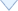/

 〈〉